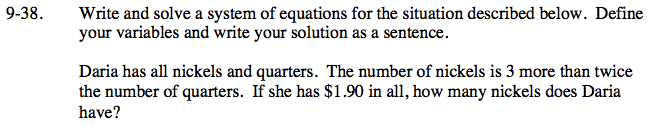### Home > CCA > Chapter 9 > Lesson 9.1.4 > Problem9-38

9-38.

Write and solve a system of equations for the situation described below. Define your variables and write your solution as a sentence. Homework Help ✎

Daria has all nickels and quarters. The number of nickels is 3 more than twice the number of quarters. If she has $1.90 in all, how many nickels does Daria have?n = number of nickels q = number of quarters so: n = 2q + 3 and$0.05n + $0.25q =$1.90.